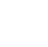Back to Chapter
Getting StartedBasic Syntax & DeclarationControl StatementsNo items found.
Function & EventsNo items found.
Array and its TypesNo items found.
OOP : Object Oriented ProgrammingNo items found.
Javascript StandardsNo items found.
HTML DOMNo items found.No items found.No items found.No items found.

# Question

Input marks (using prompt) of 3 subjects of 3 Students (By using Loop) Physics, Chemistry, Mathematics, Result their percentage and Grade according to the Following Data.

Percentage >= 90% : Grade A

Percentage >= 80% : Grade B

Percentage >= 70% : Grade C

Percentage >= 60% : Grade D

Percentage >= 40% : Grade E

Percentage < 40% : Grade F

# Hint

Make a Loop (For loop or While Loop) and take 3 Inputs using Prompt as their marks.

Then, calculate percentage of the 3 subjects from the total of 100 marks.

and use if.. Else condition to output the Grades.

# Solution

``` ```jsx var i, no1, no2, no3; for(i=1;i<=3;i++){ no1 = prompt("Enter Physics Marks"); no2 = prompt("Enter Chemistry Marks"); no3 = prompt("Enter Maths Marks"); totalMarks = parseInt(no1) + parseInt(no2) + parseInt(no3); percentage = (totalMarks / 300)*100; if((percentage <= 100) && (percentage >= 90)){ console.log("Grade is : A"); }else if((percentage < 90) && (percentage >= 80)){ console.log("Grade is : B"); }else if((percentage < 80) && (percentage >= 70)){ console.log("Grade is : C"); }else if((percentage < 70) && (percentage >= 60)){ console.log("Grade is : D"); }else if((percentage < 60) && (percentage >= 40)){ console.log("Grade is : E"); }else if((percentage < 40) && (percentage >= 0)){ console.log("Grade is : F"); }else{ console.log(" Percentage is Not Valid "); } } //Input // 90,95,100 // 80,90,80 // 70,80,70 //Output // Grade is A // Grade is B // Grade is C ``` ```